## TN State Board 11th Computer Science Important Questions Chapter 12 Arrays and Structures

Question 1.
Write the syntax to declare a one-dimensional array with example.
Syntax:
<data type><array_name> [<array_ size>];
data_type declares the basic type of the array, which is the type of each element in the array.
array name specifies the name with which the array will be referenced.
array size defines how many elements the array will hold. Size should be specified with square brackets [ ].
Eg: int num;

Question 2.
What do you mean by subscript?
Each element (Memory box) has a unique index number starting from 0 which is known as “subscript”. The subscript always starts with 0 and it should be an unsigned integer value. Each element of an array is referred by its name with subscript index within the square bracket. For example, num refers to the 4th element in the array.Question 3.
Write the formula to allocate memory space for an array.
The memory space allocated for an array can be calculated using:
Number of bytes allocated for type of array × Number of elements.

Question 4.
What do you mean by initialization of an array?
An array can be initialized at the time of its declaration. Unless an array is initialized, all the array elements contain garbage values.
Syntax:
<datatype> <array_name> [size]
= {value-1, value-2, ………, value-n};
Eg:
int age={19,,21,16,1,50};

Question 5.
How will you accept values to an array during run time?
Multiple assignment statements are required to insert values to the cells of the array during runtime. The for loop is ideally suited for iterating through the array elements.Question 6.
What do you mean by searching in a one dimensional array?
Searching is a process of finding a particular value present in a given set of numbers. The linear search or sequential search compares each element of the list with the value that has to be searched until all the elements in the array have been traversed and compared.

Question 7.
Write the syntax to initialize the character array with example.
The character array can be initialized at the time of its declaration. The syntax is shown below:
char array_name [size] = {list of characters separated by comma or a string} ;
Eg:
char country=”INDIA”;
At the end of the string, a null character is automatically added by the compiler.

Question 8.
How will you read a line of text?
cin.get( ) is used to read a line of text including blank spaces.
getline( ) is also used to read a line of text from the input stream.Question 9.
How will you access array elements?
Array elements can be used anywhere in a program as in case of a normal variable. The elements of an array are accessed with the array name followed by the subscript index within the square bracket.
Eg:
cout<<num;
In the above statement, num refers to the 4th element of the array and cout statement displays the value of num.

Question 10.
What is two dimensional array? Give syntax to declare the two dimensional array.
Two-dimensional (2D) arrays are collection of similar elements where the elements are stored in certain number of rows and columns. An example m x n matrix where m denotes the number of rows and n denotes the number of columns is shown in Figure below.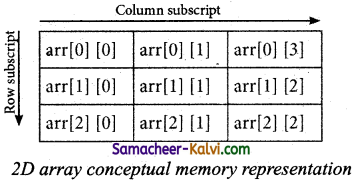The array arr can be conceptually viewed in matrix form with 3 rows and columns. Point to be noted here is since the subscript starts with 0 arr  represents the first element.
The declaration of a 2-D array is
data-type array_name[row-size] [col-size];Question 10.
Write down the bytes that are allocated for each data type.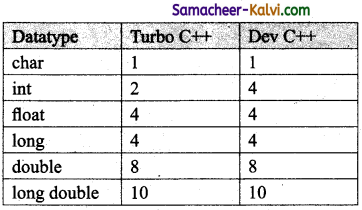Question 11.
Write a simple program to access the array elements.
#include<iostream>
using namespace std;
int main( )
{
int num = {10, 20, 30, 40, 50};
int t=2;
cout<<num <<endl; //SI cout«num [ 3+1 ] <<endl; // S2
cout<<num[t=t+l] ; // S3
Output:
30
50
40

Question 12.
Write a C++ program to read and write the values from an array.
#include<iostream>
using namespace std;
int main( )
{
int age;//declaration of array
cout<<“Enter the age of four persons:” <<endl;
for(int i=0; i<4; i++)//loop to

write array elements
cin>> age[i];
cout<<“The ages of four persons are:”;
for(int j=0; j<4; j++)
cout<< age[j]<<endl;
}Question 13.
#include <iostream>
using namespace std;
int main( )
{
int num, even=0, odd=0;
for (int i=0; i<10; i++)
{
cout<< “\n Enter Number”<< i+1
cin>>num[i];
if (num[i] %2 = = 0)
++even;
else
++odd;
}
cout<<“\n There are”«even«”Even Numbers”;
cout<<“\n There are”« odd «”Odd Numbers”;
}
Rewrite the above coding with the conditional operator instead of if.
#include<iostream>
using namespace std;
int main( )
{
int num,even=0,odd=0;
for (int i=0;i<10;i++)
{
cout<<“\n Enter Number*'<<i+1 <<“=”;
cin>>num[i] ;
(num[i]%2 = = 0)? ++even
}
cout<<“\n There are”<<even<< “Even Numbers”;
cout<<“\n There are”<<odd<<“0dd Numbers”;
>
}
Output:
Enter Number 1= 78
Enter Number 2= 51
Enter Number 3= 32
Enter Number 4= 66
Enter Number 5= 41
Enter Number 6= 68
Enter Number 7= 27
Enter Number 8= 65
Enter Number 9= 28
Enter Number 10= 94
There are 6 Even Numbers
There are 4 Odd NumbersQuestion 14.
Write a C++ program to read the prices of 10 products in an array and then print the sum and average of all the prices and also find the product of the prices.
#include<iostream>
using namespace std;
int main( )
{
float price  , sum=0, avg=0, prod=1;
for(int i=0; i<10; i++)
{
cout<<“\n Enter the price of item”<<i+1<<“=”;
cin>>price[i];
sum+=price[i];
}
avg=sum/10.0;
cout<<“\n Sum of all prices:”<<sum;
cout<<“\n Average of all prices:” <<avg;
}

Question 15.
Write a C++ program to accept the sales of each day of the month and print the total sales and average sales for each month.
#include<iostream>
using namespace std;
int main( )
int days;
float sales  , avgSales=0, totalSales=0; .
cout<<“\n Enter No. of days:”;
cin>>days;
for(int i=0; i<days; i++)
{
cout<<“\n Enter sales on day”<<i+1<<“:”;
cin>>sales [i} ;
totalSales+=sales[i];
}
avg=total sales/days;
cout<<“\n Average Sales =” <<avgSales;
return 0;
}Question 16.
Write a note on character array creation in C++.
To create any kind of array, the size (length) of the array must be known in advance, so that the memory locations can be allocated according to the size of the array. Once an_array is created, its length is fixed and cannot be changed during run time. This is shown in figure below.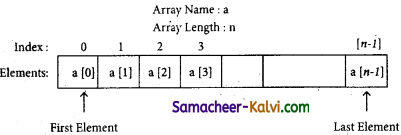Syntax:
Array declaration is:
char array_name[size];
In the above declaration, the size of the array must be. an unsigned integer value.
Eg:
char country;
The array reserves 6 bytes of memory for storing a sequence of characters. The length of the string cannot be more than 5 characters and one location is’ reserved for the null character at the end.
//Program to demonstrate a character array.
#include<iostream>
using namespace std;
int main( )
{
char country;
cout<<“Enter the name of the country:”;
cin>>country;
cout<<“The name of the country is”<<country;
}
Output:
Enter country the name: INDIA
The country name is INDIA

Question 17.
Write a C++ program to demonstrate various methods of initializing the character array.
#include<iostream>
using namespace std;
int main( )
{
char arr1=”INDIA”;
char arr2={‘I’,’N’,’D’,’I’, ‘AV\0’f;
char arr3[ ]=”TRICHY”;
char arr4[ ]={‘T’,’R’, ‘I’, ‘C’,’H’, ‘Y’, ‘\0’};
char arr5=”TRICHY”;
cout<<“arrl is :” <<arr1<< “and its size is”
<<sizeof (arr1) <<endl;
cout<<“arr2 is :” <<arr2<< “and its size is”
<<sizeof (arr2)<<endl;
cout<<“arr3 is :” <<arr3<< “and its size is”
<<sizeof (arr3) <<endl;
cout<<“arr4 is: “<<arr4<<” and its size is”
<<sizeof (arr4) <<endl;
cout<<“The elements of arr5″<<endl;
for(int i=0; i<8; i++) cout<<arr5 [i]<<” “;
return 0;
}
Output:
arr1 is :INDIA and its size is 6
arr2 is :INDIA and its size is 6
arr3 is :TRICHY and its size is 7
arr4 is :TRiCHY and its size is7
The elements of arr5 T R I C H Y.Question 18.
Write a C++ program to display a line of text using get ( ) function.
// str10.cpp
// To read a line of text
#include<iostream>
using namespace std;
int main( )
{
char str;
cout<<“Enter a string:”;
cin.get(str,100);
cout<<“You entered: “<<str<<endl;
return 0;
}
Output:
Enter a string: I am a student
You entered: I am a student

Question 19.
Write the ways to initialize the Two-Dimensional array.
The array can be initialized in more than one way at the time of 2-D array declaration.
Eg:
int matrix={
{10,20,30},// Initializes row 0
{40,50,60},// Initializes row 1
{70,80,90},// Initializes row 2
{100,110,120}// Initializes row 3
};
int matrix={10, 20, 30, 40, 50, 60, 70, 80, 90, 100, 110, 120};

Question 20.
Write a program to demonstrate array of string using 2d character array.
#include<iostream>
using namespace std;
int main ( )
{
// initialize 2d array
char colour   = {“Blue”, “Red”,”Orange”,”yellow”};
// printing strings stored in 2d array
for(int i=0; i<4; i++)
cout<<colour [i]<<“\n”;
}
Output:
Blue
Red
Orange
YellowQuestion 21.
Write a C++ program to display marks of 5 students using one dimensional array.
#include<iostream>
using namespace std;
void display (int m);
int main( )
{
int marks={88,76,90,61,69};
display(marks);
return 0;
}
void display (int m)
{
cout<<“\n Display Marks : “<<endl;
for (int i=0; i<5; i++)
{
cout<<“Student”<<i+K<“: “<<m[i]<<endl;
}
}
Output:
Display Marks:
Student 1: 88
Student 2: 76
Student 3: 90
Student 4: 61
Student 5: 69

Question 22.
How will you represent two-dimensional array?
The two-dimensional array can be viewed as a matrix. The conceptual view of a 2-D array is shown below:
int A  ;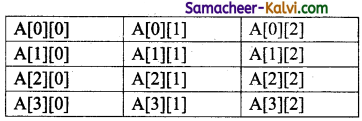In the above example, the 2-D array name A has 4 rows and 3 columns. Like one-dimensional, the 2-D array elements are stored in continuous memory.
There are two types of 2-D array memory representations. They are:
(i) Row-Major order
(ii) Column-Major order.
Eg:
int A={ .
{ 8,6,5},
{2,1,9},
{3,6,4},
{4,3,2},

Row Major order:
In row-major order, all the elements are stored row by row in continuous memory locations, i.e., all the elements in first row, then in the second row and so on. The memory representation of row major order is as shown below;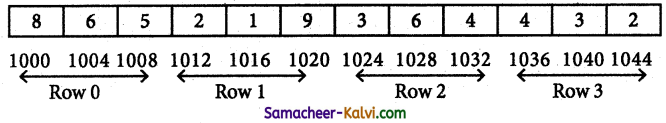Column-Major order: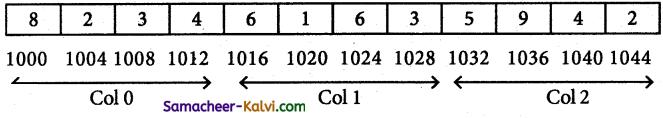Question 23.
Write a C++ program to check the given string is palindrome or not.
#include<iostream>
using namespace std;
int main( )
{
int i,j,len,flag =1;
char a ;
-cout<<“Enter a string:”;
cin>>a;
for(len=0; a[len]!-‘\0’; ++len)
for(!=0, j =len-1; i<leh/2; ++i, –j)
{
if(a[j]!=a[i])
flag=0;
}
if (flag==1) ;
cout<<“\n The String is palindrome”;
else
cout<<“\n The String is not palindrome”;
return 0;

Output:
The String is palindrome

Question 24.
Explain the returning structures from functions with example.
A structure can be passed to a function through its object. Passing a structure to a function or passing a structure object to a function is the same because structure object represents the structure. Like a normal variable, structure variable (structure object) can be passed by value or by references / addresses.

Sitnilar to built-in data types, structures also can be returned from a function.
#ihclude<iostream.h>
using namespace std;
struct Employee
{
int Id;
char Name 125];
int Age;
long Salary;
};
Employee Input( );
void main ( )
{
Employee e;
Emp = Input( );
cout<<“The values Entered are” <<endl:
cout<<“\nEmployee Id: “<<e. Id”;
cout<<“\nEmployee :”<<e.Name;
cout<<“\nEmployee Age:”<<e.Age;
cout<<“\nEmployee Salary :”<<e.Salary;
}
Employee Input( )
{
Employee e;
cout<<“\nEnter Employee Id
cin>>e.Id;
cout<<“\nEnter Employee Name :”;
cin>>e.Name;
cout<<“\nEnter Employee Age :”;
cin>>e.Age;
cout<<“\nEnter Employee Salary :”;
cin>>e. Salary;
return;
}
Output:
Enter Employee Id : 10
Enter Employee Name : Ajay
Enter Employee Age : 25
Enter Employee Salary : 15000
Employee Id : 10
Employee Name : Ajay
Employee Age : 25
Employee Salary : 15000Question 25.
Write a program to perform addition of two matrices.
#include<iostream>
#include<conio>
using namespace std;
int main ( )
{
int row, col,m1, m2,sum;
cout<<“Enter the number of rows: “;
cin>>row;
cout<<“Enter the number of columns: “;
cin>>col;
cout<<“Enter the elements of first matrix: “<<endl;
for(int i=0; i<row; i++)
for(int j=0; j<col; j++)
cin>>m1 [i] [ j ] ;
cout<<“Enter the elements of second matrix: “<<endl;
for (int i=0; i<row; i++)
for(int j=0; j<col; j++)
cin>>m2[i] [j];
cout<<“Output: “<<endl;
for(int i=0; i<row; i++)
for (int j=0; j<col; j++)
{
sum[i] [j]=ml[i] [j]+m2[i] [j];
cout<<sum [i] [ j ] <<” “;
}
cout<<endl<<endl;
}
getch( );
return 0;
}
Output:
Enter the number of rows : 2
Enter the number of column : 2
Enter the elements of first matrix:1 1 1 1
Enter the elements of second matrix:1 1 1 1
Output:
2 2
2 2

Question 26.
Write a program to accept the marks of 10 students and find the average, maximum and minimum marks.
#include<iostream>
using namespace std;
int main( )
{
int mark,i,max,min,sum=0;
float avg =0.0;
cout<<“Enter the marks:”;
for(i=0;i<10;i++)
{
cin>>mark[i];
sum += mark[i];
}
avg = sum/10;
max = mark ;
for(i=0; i<10; i++)
{
if(max<mark[i])
max = mark[i];
}
min = mark;
for(i=0; i<10; i++)
{
if(min>mark[i] )
min = mark[i];
}
cout<<“The average marks :”<<avg;
cout<<“\n The maximum mark:” <<max;
cout<<“\n The minimum mark:” <<min;
}Question 27.
Write a program to accept rainfall recorded in four metropolitan cities of India and find the city that has the highest and lowest rainfall.
#include<iostream>
using namespace std;
int main( )
{
double rainfall,sum=0,avg, highest=0,lowest;
int highest_city,lowest_city;
for (int i=0; i<4; i++)
{
cout<<“\n The total rainfall for”<<i+1<<“city:”;
cin>>rainfall [i] ;
while(rainfall[i]< 0)
{
cout<<“\n Error. Enter a positive value:”;
cout<<“\n Enter the total rainfall”<<i+1<<“city:”;
cin>>rainfall [i] ;
}
for (int x=0;x<4;x++)
{
if(highest<rainfall[x];
{
… highest = rainfall[x];
highest_city = x+1;
}
}
lowest = rainfall;
for(int y=0; y<4; y++)
{
if(lowest>rainfall[y];
lowest_city = y+1;
}
}
cout<<“\n The city has the highest rainfall is”<<highest_city<<endl;
cout<<“\n The city has the lowest rainfall is”<<lowest_ city<<endl;
return 0;
}

Question 28.
Survey your neighbouring shops and find the price of any particular product of interest and suggest where to buy the product at the lowest cost.
You are living in the heart of the city. You are very much fond of ice-creams. There are many ice-cream parlours in
your area. You want quality based product and at the same time it should be pocket-friendly.
You have collected pamphlets from three icecream outlets and the same is given below: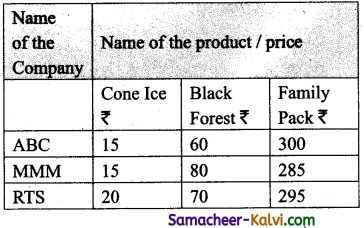Suggestions:
(i) If you want to buy cone ice cream you can buy at ABC or MMM outlets.
(ii) Black forest is cheap at ABC ice cream parlour.
(iii) MMM ice cream shop offers the lowest price for the family pack.Question 29.
What is Traversal in an Array?
Accessing each element of an array at least once to perform any operation is known as “Traversal”.

Question 30.
What is Strings?
A string is defined as a sequence of characters where each character may be a letter, number or a symbol. Each element occupies one byte of memory. Every string is terminated by a null (‘\0’, ASCII code 0) character which must be appended at the end of the string.

Question 31.
What is the syntax to declare two – dimensional array.
data-type array_name[row-size][col-size];
Eg: int A ;

Question 32.
Define an Array? What are the types?
“An array is a collection of variables of the same type that are referenced by a common name”.
Types of Arrays:

1. One-dimensional arrays
2. Two-dimensional arrays
3. Multi-dimensional arraysQuestion 33.
Write note on Array of strings.
An array of strings is a two-dimensional character array. The size of the first index (rows) denotes the number of strings and the size of the second index (columns) denotes the maximum length of each string. Usually, array of strings are declared in such a way to accommodate the null character at the end of each string. For example, the 2-D array has the declaration:
char N ame ;
In the above declaration, the 2-D array has two indices which refer to the row size and column size, that is 6 refers to the number of rows and 10 refers to the number of columns.

Question 34.
Write a C++ program to accept and print your name?
#include<iostream>
using namespace std;
int main ( )
{
char name;
cin>>name;
}
Output:

Question 35.
Write a C++ program to find the difference between two matrix.
#include<iostream>
#include<conio.h>
using namespace std;
int main( )
{
int row,col,ml,m2, diff  ;
cout«”Enter the number of rows:”;
cin>>row;
cout<<“Er,ter the number of columns:”; cin»col;
cout<<“Enter the elements of first matrix:”«endl; for(int i-0;icrow;i++) .
for(int j=0; j<col; j++)
{
cin>>m1 [i] [ j ] ;
}
}
cout<<“Enter the elements of ; second matrix: “<<endl;
for(int i=0; i<row; i++)
for(int j=0; j<col; j++)
{
cin>>m2[i] [ j];
}
}
cout<<“output: “<<endl;
for(int i=0; i<row; i++)
{
for(int j=0; j<col; j++)
{
diff [ i ] [ j ] = m1 [i][ j] – m2 [i][j];
cout<<diff[i] [j]<< ” “;
}
cout<<endl<<endl;
}
return 0;
}
Output:
Enter the number _ of rows : 2
Enter the number of columns : 2
Enter the elements of first matrix :
3
3
3
3
Enter the elements of second matrix :
1 .
1
1
1
Output:
2 2
2 2Question 36.
How will you pass two dimensional array to a function explain with example.
In C++, array can be passed to a function as an argument. To pass an array to a function in C++, the function needs the array name as an argument.
C++ program to display values from two dimensional array:
#include<iostream>
using namespace std;
void display(int n);
int main ( )
{
int num = {{3,4}, {9,5}, {7,1}};
display(num);
return 0;
}
void display(int n)
{
cout<<“\n Displaying Values” <<endl;
for (int i=0; i<3; i++)
{
for(int j=0; j<2; j++)
{
cout«n [i] [ j ] <<”
}
cout<<endl<<endl;
}
}
Output:
Displaying Values
3 4
9 5
7 1

Question 37.
Define structure. What is its use?
Structure is a user-defined which has the combination of data items with different data types.
Uses:
This allows to group of variables of mixed data types together into a single unit.

Question 38.
To store 100 integer number, Which of the following is good to use? Array or Structure. State the reason.
In any situation, when more than one variable is required to represent objects of uniform data-types array can be used. Because it is used to store number of same data type in sequential manner. It provides an easy access to element due to the usage of index number.Question 39.
What is the error in the following structure definition?
struct employee{inteno; charename; char dept;}
Employee e1,e2;

 Incorrect statement Correct statement inteno; intno; char ename char name; char dept; char dept

Question 40.
Write a structure definition for the structure student containing examno, name and an array for storing five subject marks.
struct student
{
int examno;
char name;
int marks;
};

Question 41.
Why for passing a structure to a function, call by reference is advisable to us?
Structures are usually passed by reference method because it saves the memory space and executes faster.Question 42.
What is the size of the following highlighted variable in terms of byte if it is compiled in dev C++ ?
struct A{ float f; char ch;
long double d;};
struct B{ A a; int arr;}b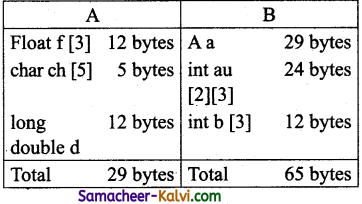Question 43.
Is the following snippet is fully correct. If not identify the error.
struct sum1{int nl,n2;}s1;
struct sum2{int nl,n2}s2;
cin>>s1 .n1>>s1 .n2;
s2=s1;
The error statement is s2 = s1;
The object name must be followed with a dot (.) and the member name.

Question 44.
Differentiate array and structure.

 Array Structures Array is a collection of variables of similar data type. Structure is a collection of variables of dissimilar data types. Variables of an array are stored in a contiguous memory location. The variables in a structure may or may not be stored in a contiguous memory locations. Array elements are accessed by their index number. Structure elements are accessed by their names.Question 45.
What are the different ways to initialize the structure members?
Values can be assigned to structure elements similar to assigning values to variables.
Eg:
balu.rollno= “702016”;
balu.age= 18;
balu.weight- 48.5;
Also, values can be assigned directly as similar to assigning values to Arrays.
balu={702016,18,48.5};

Question 46.
What is wrong with the following C++ declarations?
A. struct point ( double x, y)
B. struct point (double x, double y };
C. struct point {double x; double y}
D. struct point {double x; double y;};
E. struct point { double x; double y;}
struct point {double x; double y;}; is the only one syntactically correct.

Question 47.
How will you pass a structure to a function?
A structure variable can be passed to a function in a similar way of passing any argument that is of built-in data type.

1. If the structure itself is an argument, then it is called “call by value”.
2. If the reference of the structure is passed as an argument then it is called, “call by reference”.Question 48.
The following code sums up the total of all students name starting with ‘^S’ and display it. Fill in the blanks with required statements.
struct student {int exam no, lang, eng, phy, che, mat, csc, total;char name;};
int main( )
{
student s;
for(int i=0;i<20;i++)
{
//accept student details
}
for(int i=0;i<20;i++)
{
//check for name starts with letter “S”
// display the detail of the checked name
}
return 0;
}
#include<iostream>
using namespace std;
struct student
{
int examno, lang, eng, phy, che, mat, esc, total;
Char name;
};
int main ( )
{
student s ;
for(int i=0; i<20; i++)
{
cout<<“\n Enter Exam no”;
cin>>examno;
cout<<“Enter Name”
cin>>name;
cout<<“\n Enter language”;
cin>>lang;
cout<<“\n Enter English mark”;
cin>>eng;
cout<<“\n Enter Physics mark”;
cin>>phy;
cout<<“\n Enter Chemistry mark”;
cin>>che;
cout<<“\n Enter Maths mark”;
cin>>mat;
cout<<“\n Enter CSC mark”;
cin»csc; .
}
cout<<“Displaying the information the name starts
Now the elements of the structure student can be accessed as follows.
balu.rollno
balu.age
balu.weight
frank.rollno
frank.age
frank.weight.

Question 49.
Write the syntax and an example for structure.
Syntax:
struct structure_name
{
type member_namel;
type member_name2;
} reference_name;
An optional field reference name can be used to declare objects of the structure type directly.
Eg:
struct Student
{
long rollno;
int age;
float weight;
};Question 50.
For the following structure definition write the user defined function to accept data through keyboard.
struct date{ int dd,mm,yy};
struct item {int item id; char name;float price;
date date_manif;}
{
cout<<“Enter date”;
cin>>d.dd;
cout<<“Enter month”;
cin>>d.mm;
cout<<“Enter year”;
cin>>d;yy;
}
{
cout<<“Enter Item code:”;
cin>>i.item_id;
cout<<“Enter Name:”; cin»i.name;
cout<<“Enter Price:”;
cin>>i .price;
cout<<“Enter date of manufacture:”;
cin>>i. date_manif;
}

Question 51.
What is called anonymous structure? Give an example.
Anonymous Structure A structure without a name/tag is called anonymous structure.
struct
{
long rollno;
int age;
float weight;
} student;
The student can be referred as reference name to the above structure and the elements can be accessed like student.rollno, student, age and student.weight.

Question 52.
Write a user defined function to return the structure after accepting value through keyboard. The structure definition is as follows:
struct ltem{int item no;float price;};
item Input( )
{
item I
cout<<“Enter Item Number”;
cin>>I.itemno;
cout<<“Enter Price”;
cin>>Price;
return;
}Question 53.
Explain array of structures with example.
An array of structures is declared in the same way as declaring an array with built-in data types like int or char.
The following program reads the details of 20 students and prints the same.
#include<iostream>
using namespace std;
struct Student
{
int age;
float height,weight; char name;
with letters”;<<endl;
for(int i=0; i<=3; i++.)
{
if(s[i].name[0} == ‘S’
{
cout<<s[i] .examno<<endl;
cout<<s [i].name<<endl;
cout<<s [i]. language<<endl;
cout<<s [i].Eng<<endl;
cout<<s [i].Phy<<endl;
cout<<s[i].Che<<endl;
cout<<s [i] .Mat<<endl;
cout<<s [i] .Csc<<endl;
}
}
return 0;
}

Question 54.
What is called nested structure? Give example.
The structure declared within another structure is called a nested structure.
Eg:
The following code the struct date of birth can be assigned as one of the elements in the student structure.

Now the student structure will be,
struct Student
{
int age;
float height, weight;
struct dob
{
int date;
char month;
int year;
};
};Question 55.
Rewrite the following program after removing the syntactical error(s),if any.
Underline each correction.
struct movie
{
charm_name;
charm_lang;
float ticket cost =50;};
Movie;
void main( )
{
gets(m_name);
cin>>mjang;
return 0;
}
struct movie
{
char m_name;
char m_lang;
float ticket_Cost =50;
}movie;
int main( )
{
gets(movie.m_name);
cin>>movie.m lang;
return 0;
}

Question 56.
What is the difference among the following two programs?
(a) #inelude <iostream.h>
struct point { double x; double y;};
int main( ) {
struct point test;
test.x = .25; test.y = .75;
cout<<test.x<<test.y;
return 0;
}

(b) #include <iostream.h>
struct { double x; double y;} Point;
int main(void) {
Point test={.25;. 75};
return 0;
}
The program (b) is the anonymous structure.
The program (b) does not contain a name/tag in the structure.Question 57.
How to access members of a structure? Give example.
The members of the structure can be accessed directly by using a dot(.)
Eg:
struct Student
{
long rollno;
int age;
float weight;
}balu,frank;
};
void main( )
{
Student std;
int i;
Cout<<“Enter the details for 20 students”<<endl;
for(i=0; i<20; i++)
{
cout<<“Enter the details of Student”<<i+1<<endl;
cout<<“Enter the age:”<<endl;
cin>>std[i].age;
cout<<“Enter the. height: “<<endl;
cin>>std[i] .height;
cout<<“Enter the weight: “<<endl;
cin>>std[i] .weight;
}. . .
cout<<“The values entered for Age,.height and weight are”<<endl;
for(i=0; i<20; i++)
cout<<“Student”<<i+l<<“\t”<<std[i] .age<<“\t”<<std[i] . height<< “\t”<<std [i] weight;
}
Output:
Enter the details of 20 students details for students age:
height:
weight:
details for student2 age:
height:
Enter -the weight: 61.5
The values entered for Age, height and weight are Student 1 18 160.5 46.5 Student 2 18 164-5 61.5

Question 58.
Explain call by value with respect to structure.
When a structure is passed as argument to a function using call by vatue method, any change made to the contents of the structure variable inside the function to which it is passed do not affect the structure variable used as an argument.
Eg:
#include<iostream>
using namespace std;
struct Employee
{
char.name;
int age;
float salary;
};
void printData(Employee);
//Function declaration
int main( )
{
Employee p;
cout<<“Enter Full name:”;
cin>>p.name;
cout<<“Enter age:”;
cin>>p.age;
cout<<“Enter salary:”;
cin>>p. salary;
// Function call with structure variable as an argument printData(p);
return 0;
}
void printData(Employee q)
{
cout<<“\nDi splaying Information.” <<endl;
cout<<“Name: “<< q.name <<endl;
cout<<“Age: “<<q. age<<endl;
cout<<“Salary: “<<q. salary;
}
Output:
Enter Full name: Kumar
Enter age: 55
Enter salary: 34233.4
Displaying Information.
Name: Kumar
Age: 55
Salary: 34233.4
In the above example, a structure named Employee is declared and used. The values that are entered into the structure are name, age and salary of a Employee are displayed using a function named printDataQ. The ^ argument for the above function is the structure Employee. The input ban be received through a function named readData( ).Question 59.
How call by reference is used to pass structure to a function. Give an Example.
In this method of passing the structures to functions, the address of a structure variable
/object is passed to the function using address of(&) operator. So any change made to the contents of structure variable inside the function are reflected back to the calling function.
Eg:
#include<iostream>
using namespace std;
struct Employee
{
char name;
int age;
float salary;
};
void printData(Employee);
int main( )
{
Employee p;
printData (p);
return 0;
}

{
cout<<“Enter Full name:”;
cin.get(p.name, 50) ;
cout<<“Enter age:”;
cin>>p. age;
cout<<“Enter salary:”;
cin>>p.salary;
}
void printData(Employee p)
{
cout<<“\nDisplaying Information. “<<endl;
cout<<“Name: “<<p.name<<endl;
cout<<“Age: “<<:p. age<<endl;
Cout<<“Salafy: “<<p. salary;
}
Output:
Enter Full name: Kumar
Enter age: 55
Enter Salary: 34233.4
Displaying Information.
Name: Kumar
Age: 55
Salary: 34233.4

Question 60.
Write a C++ program to add two distances using the following structure definition.
Struct Distance
{
int feet;
float inch;
}
d1 , d2, sum;
PROGRAM:
#include<idstream>
using namespace std;
Struct Distance .
{
int feet;
float inch; .
}
d1, d2, sum;

int main( )
{
cout<<“Enter 1st distance”<<endl;
cout<<“Enter feet:”;
cin>>d1.feet;
cout<<“Enter inch:”
cin>>d1.inch;
cout<<“nEnter information for 2nd distance”<<endl;
Cout<<“Enter feet:”; ‘
cin>>d2. feet;
cout<<“Enter inch:”;
cin>>d2.inCh;
sum.feet = d1.feet + d2.feet;
sum.inch = d1.inch + d2.inch;
//Changing to feet if inch is greater than 12
if(sum.inch>12)
{
++ sum.feet;
sum.inch – = 12;
}
cout<<endl<<“sum of distances-“<<sum. feet<<“feet”<<sum.inch<<“inches”;
return 0;
}
Output:
Enter 1st .distance
Enter feet : 6
Enter inch : 3.4
Enter infdtmatidh for 2nd distance
Enter feet : 5
Enter inch : 10.2
Sum of distances – 12 feet 1.6 inchesQuestion 61.
Write a C++ Program to Add two Complex Numbers by Passing Structure to a Function for the following structure definition,
struct complex
{
float real;
float imag;
};
The prototype of the function is complex add Complex Numbers(complex, complex);
#include<iostream>
using namespace std;
typedef struct complex
{
float real;
float, imag;
}
Complex Number;
int main( )
{
Complex Number n1, n2, temporaryNumber;
char SignofImag;
cout<<“For 1st complex number,”<<endl;
cout<<“Enter real and imaginary parts respectively:”<<endl;
cin>>n1. real>>n2 . imag;
cout<<“For 2nd complex number “<<endl;
cout<<“Enter real and imaginary parts respectively:”<<endl;
cin>>n2 . real>>n2 . imag;
SignofImag =(temporaryNumber. , imag>0) ? ‘+’: ‘-‘;
cout<<“Ehter real and imaginary parts respectively:”<<endl;
cin>>n2 . real>>n2 . imag;
SignofImag =(temporaryNumber. , imag>0) ?
temperaryNumber. imag = (temporaryNurnbet.imag>0)? temporaryNumber .imag: – temporaryNumber.imag; temporaryNumber = addComplexNumbers(n1,n2);
cout<<“Sum = ” <<temporaryNumber. real<<temporatyNumber. imag<<“i”;
return 0;
}
ComplexNumber
{
complex temp;
temp.real – n1.real + n2.real;
temp.imag = hi. imag + n2. imag;
return(temp);
}
Output:
For 1st complex number,
Enter real and imaginary parts respectively:
3.4
5.5
For 2nd complex number,
Enter real and imaginary parts, respectively:
– 4.5
– 9.5
sum = -1.1 – 4iQuestion 62.
Write a C++ Program to declare a structure book containing name and author as character array of 20 elements each and price as integer. Declare an array of book. Accept the name, author, price detail for each book. Define a user defined function to display the book details and calculate the total price. Return total price to the calling function.
#inciude<iostream>
using namespace std;
struct Book
{
char bookname ;
char author;
float price;
}b ;
void displaydata(Book);
int main( )
{
for (int i=1; i<-3; i++)
(
cout<<“Enter Book Name :”;
cin>>b [ i ] .bookname;
Gout<<“Enter Author Name :”;
cin>>b[i] .author;
cout<<“Enter Price :”;
cin>>b [i] .price;
}
displaydata (b. ) ;
return 0;
}
void displaydata(Book)
float total=0.0;
cout<<“Displaying Book Details”<<endl;
cout<<“\nS.No\tBook Name\tAuthor Name\tPrice”<<endl;
for(int i=1;i<=3;i++)
{
cout<<i<<“\t”<<b [i] .bookname<<“\t”<<b [i] . author<<“\t”<<b [i] .
price<<endl;
total+=b[i].price;
}
cout<<“\nThe total price =”<<total<<endl;
}
Output:
Enter Book Name :Programming
Enter Author Name :Dromy
Enter Price :150
Enter Book Name:C++programming
Enter Author Name :BjarneStrouStrup
Enter Price :200
Enter Book Name :JavaProgramming
Enter Author Name :James
Enter Price :220
Displaying Book Details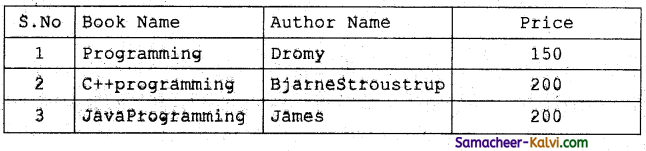The Total Price = 550Question 63.
Write a C++ program to declare and accept an array of professors. Display the details of the department=”COMP.SCI” and the name of the professors start with ‘A’. The structure “college” should contain the following members.
prof_id as integer
name and Department as character array
#include<iostream>
#include<string.h>
using namespace std;
struct college
{
int Prof_Id;
char name;
char Department;
};
int main( )
{
college c  ;
for(int i=0; i<=3; i++)
{
cout<<“Enter Record”<i + 1<<endl;
cout<<“Enter Prof_Id”<<endl ;
cin>>c[i] . Prof_Id;
cout<<“Enter Professor Name” <<endl;
cin>>c [i] .name;
cout<<“Enter Department”<<endl ;
cin>>c[i].Department;
}
cout<<“Displaying the information”<<endl;
for(int i=0; i<=3; i++)
{
if(strcmp (c [i] .Department, “COMP.SCI”)==0)&&
(s[i].name==’A’) | | (s[i]. name==’a’)

Question 64.
Write the output of the following C++ program.
#include<iostream>
#include<stdio>
#include <string>
#include<conio>
using namespace std;
struct books
{
char name, author;
}
a ;
int main( )
{
cout<<c[i] .Prof_Id<<endl;
cout<<c[i] .name<<endl;
cout<<c[i] . Department<<endl;
}
}
return 0;
}
Output:
Enter Record 1
Enter Prof_Id
1001
Enter Name
Aarthi
Enter Department
COMP.SCI
Enter Record 2
Enter Prof_Id
1002
Enter Name
Sangeetha
Enter Department
Physics
Enter Record 3
Enter Prof_Id
1003
Enter Name Kavitha
Enter Department
Chemistry
Enter Record 4
Enter Prof_Id
1004
Enter Name
Saritha
Enter Department
Physics
Displaying the information
1001
Aarthi
COMP.SCI
clrscr( );
cout<<“Details of Book No” <, 1<, “\n”;
cout<,”————-\n”;
cout<< “Book Name :”<<strcpy(a.name,”Programming”)<<endl; ’
cout<< “Book Author :”<<strcpy(a.author,”Dromy”)<<endl;
cout<< “\nDetails of Book No”<< 2 << “\n”;
cout<< ” ———–\n”;
cout<< “Book Name :”<<strcpy(a.name,”C++programming” )<<endl;
cout<< “Book Author :”<<strcpy(a.author,”BjarneStroustrup”)<<endl;
cout<<“\n\n”;
cout<< “==================\n”;
cout<< “S.No\t| Book Name\t|author\n”;
cout<, “=================”;
for (Int i = 0; i < 2; i++)
{
cout<<“\n”<< i + 1<< “\t |” << a[i].riame << “\t |”<< a[i].author;
}
cout<< “\n=======-========”;
return 0;
}
Output:
DETAILS OF BOOK NO 1
Book Name : Programming
Book Author : Dromy

DETAILS OF BOOK NO 2
Book Name : C++ Programming
Book Author : BjarneStroustrup
=======================
S.No | Book Name | author
=======================
1 | Programming | Dromy
2 | C++ Programming | BjarneStroustrupQuestion 65.
Write the output of the following C++ program.
#include <iostream>
#include <string>
using namespace std; ,
struct student
{
int roll_no;
char name;
long phone_number;
};
int main( ){
student p1 = {1,”Brown”, 123443};
student p2, p3;
p2.roll_no = 2;
strcpy(p2.name ,”Sam”);
p2.phone_number = 1234567822;
p3.roll_no = 3; ,
p3.phone_number = 1234567844;
eout<< “First Student” <<endl;
cout,<< “roll no :”<< p1.roll_no <<endl;
cout<< “name :”<< pi.name <<endl;
cout<< “phone no :”<< p1.phone_number <<endl;
cout<< “Second Student”<<endl;
cout<< “roll no :”<< p2.roll_no <<endl;
cout<< “name :”<< p2.name <<endl;
cout<< “phone no :”<< p2.phone_number <<endl;
cout<< “Third Student”<<endl;
cout<< “roll no :”<< p3.ro|l_no <<endl;
cout<, “name :”<< p3.name <<endl;
cout<<“phone no;”<< p3.phone_number <<endl;
return 0;
}
Output:
First Student
roll no : 1
name : Brown
phone no : 123443
Second Student
roll no : 2
name : Sam
phone no : 1234567822
Third Student roll no : 3
phone no : 1234567844

Question 66.
Debug the error in the following program,
#include <is.vream.h>
structPersonRec
{
charlastName;
chaefirstName;
intage;
}
Person RecPeopleArrayType;
void main( )
{
PersonRecord people;
for (i = 0; i < 10; i++)
{
cout<<people.firstName<<” <<people. lastName
<<setw(10) <<people.age;
}
}
{
for (int i = 0; i < 10; i++)
{
cout<< “Enter first name:”;
cin<<peop[i].firstName;
cout<< “Enter last name:”;
cin>>peop[i].lastName;
cout<< “Enter age:”;
cin>> people[i].age;}
#include<iostream>
#include<iomanip.h>
using namespace std;
struct_PersonRec
{
char lastName;
char firstName ;
int age;
};
PersonRec PeopleArrayType;
int main( )
{
PeopleArrayType people;
for (int i=0;i<10;i++)
{
cout<<people [ i ] .firstName<<‘ ‘<<people(i].lastName <<setw (10) <<people[ i ] . age;
}
}
{
for (int i=0;i<10;i++)
{
cout<<“Enter first name:”;
cin>>peop[i] .firstName;
cout<<“Enter last name:”;
cin>>peop [i] . lastName;
cout<<“Enter age:”;
cin>>peop[i] .age;
}Question 1.
________ is a collection of variables of the same type that are referenced by a common name.
(a) Structure
(b) Array
(c) Function
(d) Class
(b) Array

Question 2.
There are ______ types of arrays used in C++.
(a) three
(b) four
(c) five
(d) six
(a) three

Question 3.
Displaying all the elements in an array is an example of:
(a) walk through
(b) traversal
(c) accessing
(d) statements
(b) traversalQuestion 4.
Which of the following correctly declares an array?
(a) int num;
(b) int num
(c) num{10};
(d) num num;
(a) int num;

Question 5.
What is the index number of the last element of an with 6 elements?
(a) 6
(b) 5
(c) 0
(d) 1
(b) 5

Question 6.
Which of the following gives the memory address of the first element in array?
(a) num;
(b) num[l];
(c) num(O);
(d) num( 1);
(a) num;Question 7.
Which of the following access the seventh element stored in array?
(a) num(7);
(b) num(6);
(c) num;
(d) num;
(d) num;

Question 8.
________ is a process of finding a particular value present in a given set of numbers.
(a) Finding
(b) Searching
(c) Traversing
(d) Walkthrough
(b) Searching

Question 9.
____ function receives array, size and value to be searched as parameters.
(a) find( )
(b) replace( )
(c) searching( )
(d) search( )
(d) search( )Question 10.
(a) 0
(b) 1
(c) array index
(d) -1
(d) -1

Question 11.
_____ is defined as a sequence of characters.
(a) Characters
(b) Numbers
(c) Arrays
(d) String
(d) String

Question 12.
char country;
In this array reserves _________ bytes of memory.
(a) 5
(b) 6
(c) 0
(d) none
(b) 6Question 13.
In the initialization of the string, if all the characters are not initialized, then the rest of the characters will be filled with:
(a) Zero
(b) Empty
(c) 1
(d) Null
(d) Null

Question 14.
________ is used to read a line of text including blank spaces.
(a) cin.get( )
(b) gets( )
(a) cin.get( )

Question 15.
In C++, __________ is also used to read a line of text from the input stream.
(a) getsline( )
(c) putline( )
(d) getline( )
(d) getline( )Question 16.
________ arrays are collection of similar elements where the elements are stored in certain number of rows and columns.
(a) Single-dimensional
(b) Two-dimensional
(c) Structures
(d) Functions
(b) Two-dimensional

Question 17.
Identify the invalid:
(a) int A;
(b) float x;
(c) char name;
(d) int A(3)(4);
(d) int A(3)(4);

Question 18.
An array of _______is a two-dimensional character array.
(a) character
(b) strings
(c) number
(d) symbols
(b) stringsQuestion 19.
int a declares:
(a) 3 rows and 3 columns
(b) 2 rows and 3 columns
(c) 2 columns and 3 rows
(d) 3 rows and 2 columns
(d) 3 rows and 2 columns

Question 20.
Determine the number of elements in the following declaration int array;
(a) 10
(b) 12
(c) 120
(d) 100
(c) 120

Question 21.
Structure is declared using the keyword:
(a) str
(b) structure
(c) struct
(d) std
(c) structQuestion 22.
Objects declared along with structure definition are called _______ objects.
(a) global
(b) local
(c) class
(d) function
(a) global

Question 23.
By default all members are ________ in a structure.
(a) private
(b) protected
(c) public
(d) static
(c) public

Question 24.
A structure without a name/tag is called ________
(a) anonymous
(c) infinite
(b) finite
(d) empty
(a) anonymousQuestion 25.
The structure declared within another structure is ealled a _______ structure.
(a) looping
(b) nested
(c) iterative
(d) selection
(b) nested

Question 26.
Nested structures act as _________ of another structure.
(a) objects
(b) variables
(c) members
(d) classes
(c) members

Question 27.
If the structure itself is an argument, then it is called:
(a) call by method
(b) call by value
(c) call by reference
(d) call by obj ect
(b) call by valueQuestion 28.
While declaring and initializing values in an array the values should be given within the:
(a) {}
(b) <>
(c) ( )
(d) [ ]
(a) {}

Question 29.
By default all members ar ______ in a structure.
(a) public
(b) private
(c) protected
(d) struct
(a) public

Question 30.
The array subscripts always commences from:
(a) 1
(b) 0
(c) -1
(d)10
(b) 0Question 31.
The second index of the 2D-array refers to:
(a) column
(b) row
(c) length
(d) size
(a) column

Question 32.
The header file for setw( ):
(a) iostream
(b) iomanip
(c) stdio
(d) conio
(b) iomanip

Question 33.
________ is used between the object name and the member name.
(a) .
(b) ;
(c) :
(d) ,
(a) .Question 34.
Matrices can be represented through:
(a) single dimensional array
(b) 2-D array
(c) 3-D array
(d) 4-D array
(b) 2-D array

Question 35.
In C++, array subscripts is enclosed within ______ symbol.
(a) [ ]
(b) { }
(c) ( )
(d) < >
(a) [ ]

Question 36.
The appropriate declaration statement to initialize the variable ‘name’ with the value ‘computer’ is:
(a) char name = “computer”
(b) char[ ] name = “computer”;
(c) char name[ ] – computer;
(d) char name[ ] – “computer”;
(d) char name[ ] – “computer”;Question 37.
Which of the following is an invalid array declaration?
(a) int array;
(b) int array[ ];
(c) int array[i]
(d) const int i – 10; int array[i];
(b) int array[ ];

Question 38.
How many elements are stored in an array int num?
(a) 2
(b) 4
(c) 6
(d) 8
(d) 8

Question 39.
Which function returns 0, if the strings are equal?
(a) strcmp( )
(b) strcpy( )
(c) strlen( )
(d) strcat( )
(a) strcmp( )Question 40.
Which of the following is called as literals?
(a) String
(b) Float
(c) Int
(d) Char
(a) String

Question 41.
Match the following:

 (i) Variable (a) Sequence of characters (ii) Array (b) Read a line of text (iii) String (c) Basic building blocks in C++ (iv) Getline( ) (d) Collection of variables of the same type

(a) (i) – (d); (ii) – (a); (iii) – (c); (iv) – (b)
(b) (i) – (a); (ii) – (c); (iii) – (b); (iv) – (d)
(c) (i) – (d); (ii) – (a); (iii) – (b); (iv) – (c)
(d) (i) – (c); (ii) – (d); (iii) – (a); (iv) – (b)
(d) (i) – (c); (ii) – (d); (iii) – (a); (iv) – (b)

Question 42.
Identify the correct statement:
(a) Array size must be unsigned integer value which is greater than 0.
(b) In arrays, column size is optional but row size is compulsory.
(c) An array of strings is a two-dimensional character array.
(d) Displaying all the elements is an array is an example of‘‘traversal”
(b) In arrays, column size is optional but row size is compulsory.Question 43.
Identify the correct statement:
(a) If the structure itself is an argument it is called “call by reference”
(b) If the structure is passed as an argument then it is called “call by value”
(c) Structure assignment is possible only if both structure variables / objects are same type
(d) Structure is declared using the keyword “array”
(c) Structure assignment is possible only if both structure variables / objects are same type

Question 44.
Assertion (A):
An array is a collection of variables of the same type that are referenced by a common name.
Reason (R):
In an array the values are stored in a fixed number of elements of the same type sequentially in memory.
(a) Both A and R are true and R is the correct explanation for A.
(b) Both A and R are true, but R is not the correct explanation for A.
(c) A is true, but R is false.
(d) A is false, but R is true.
(a) Both A and R are true and R is the correct explanation for A.

Question 45.
Which of the following is the collection of variables of the same type that are referenced by a common name?
(a) int
(b) float
(c) Array
(d) class
(c) ArrayQuestion 46.
Array subscripts is always starts with which number?
(a) -1
(b) 0
(c) 2
(d) 3
(b) 0

Question 47.
int age[ ] = {6,90,20,18,2}; How many elements are there in this array? ‘
(a) 2
(b) 5
(c) -6
(d) 4
(b) 5

Question 48.
cin>>n; To which element does this statement accepts the value?
(a) 2
(b) 3
(c) 4
(d) 5
(c) 4Question 49.
By default, the string end with which character?
(a) \o
(b) \t
(c)\n
(d) \b
(a) \o

Question 50.
The data elements in the structure are also known as:
(a) objects
(b) members
(c) data
(d) records
(b) members

Question 51.
Structure definition is terminated by:
(a) :
(b) }
(c) ;
(d) ::
(c) ;Question 52.
What will happen when the structure is declared?
(a) it will not allocate any memory
(b) it will allocate file memory
(c) it will be declared and initialized
(d) it will be only declared
(a) it will not allocate any memory

Question 53.
What is the output of this program?
#include<iostream>
#include<string.h>
using namespace std;
int main ( )
{
struct student
{
int n;
char name;
};
student s;
s.n – 123;
strcpy(s.name,”Balu”);
cout<<s.n;
cout<<s. name«endl;
return 0;
}
(a) 123Balu
(b) BaluBalu
(c) Balu 123
(d) 123 Balu
(a) 123BaluQuestion 54.
A structure declaration is given below,
struct Time
{
int hours; int minutes;
int seconds;
} t;
Using declaration which of the following refers to seconds.
(a) Time. seconds
(b) Time:: seconds
(c) seconds
(d) t. seconds
(d) t. seconds

Question 55.
What will be the output of this program?
#include<iostream>
using namespace std;
struct ShoeType
{
string name;
double price;
};
int main ( )
{
ShoeType shoel,shoe2;
shoel.price = 9.99;
cout<<shoel. name<<“#”<<shoe1.price<<end1;
shoe2 = shoe1;
shoe2.price = shoe2.price/9;
cout<<shoe2. name<<“#”<<shoe2.price;
return 0;

Question 56.
Which of the following is a properly defined structure?
(a) struct {intnum;}
(b) struct sum {int num;}
(c) struct sum int sum;
(d) struct sum {int num;};
(d) struct sum {int num;};Question 57.
A structure declaration is given below.
struct employee
{
int empno;
char ename;
}e  ;
Using above declaration which of the following statement is correct.
(a) cout<<e.empno<<e.ename;
(b) cout<<e.empno<<ename;
(c) cout<<e→empno<<e->ename;
(d) cout<<e.empno<<e.ename;
(a) cout<<e.empno<<e.ename;

Question 58.
Which of the following cannot be a structure member?
(a) Another structure
(b) Function
(c) Array
(d) Variable of double data type
(b) FunctionQuestion 59.
When accessing a structure member, the identifier to the left of the dot operator is the name of:
(a) structure variable
(b) structure tag
(c) structure member
(d) structure function